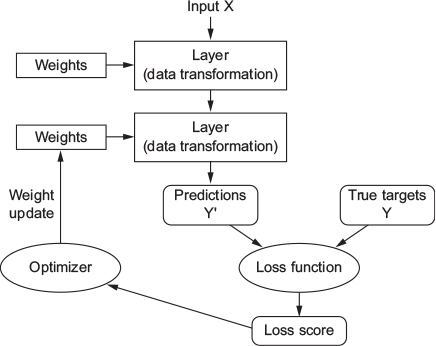## 15.9 Understanding how DL works (3)

• Loss score: Is used as feedback signal to adjust the value of the weights, in a direction that will lower loss score for the current example (see Figure 15.6)
• Adjustment = job of optimizer
• Optimizer: Implements Backpropagation algorithm (central algorithm in deep learning)
• Starting point: weights of the network are assigned random values and network implements series of random transformations
• Output far from targets and loss score is high
• Network processes examples and adjusts weights a little in correct direction → with every example loss score decreases (= training loop)
• Training loop: repeated a sufficient number of times, yields weight values that minimize the loss function
• Typically tens of iterations over thousands of examples
• Trained network: Network with minimal loss for which the outputs are as close as they can be to the targetsFigure 15.6: The loss score is used as a feedback signal to adjust the weights (Chollet & Allaire, 2018, Fig. 1.9)